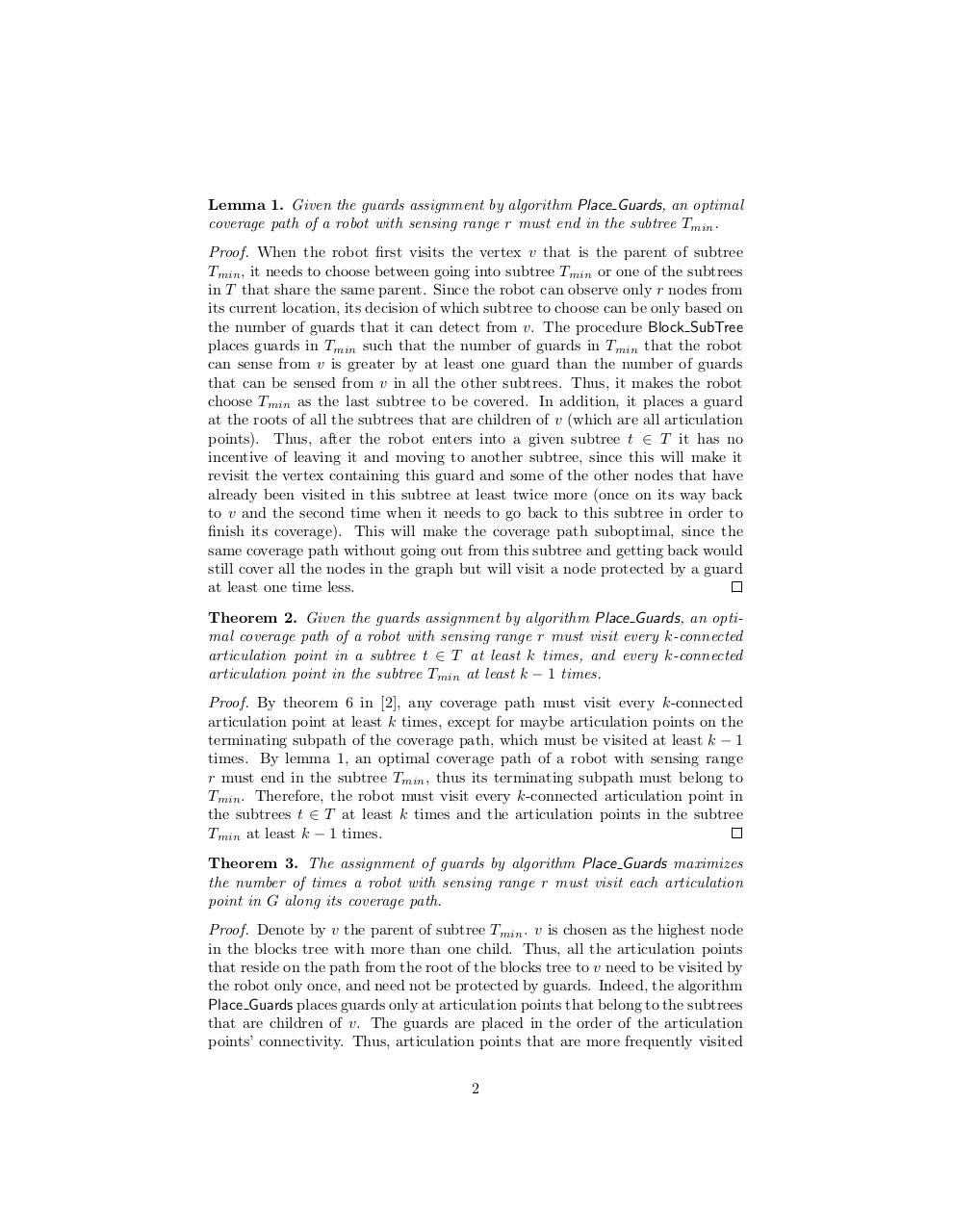Page 1 2 3 4 5 6 7 8 9 10

#### Text preview

Lemma 1. Given the guards assignment by algorithm Place Guards, an optimal
coverage path of a robot with sensing range r must end in the subtree Tmin .
Proof. When the robot first visits the vertex v that is the parent of subtree
Tmin , it needs to choose between going into subtree Tmin or one of the subtrees
in T that share the same parent. Since the robot can observe only r nodes from
its current location, its decision of which subtree to choose can be only based on
the number of guards that it can detect from v. The procedure Block SubTree
places guards in Tmin such that the number of guards in Tmin that the robot
can sense from v is greater by at least one guard than the number of guards
that can be sensed from v in all the other subtrees. Thus, it makes the robot
choose Tmin as the last subtree to be covered. In addition, it places a guard
at the roots of all the subtrees that are children of v (which are all articulation
points). Thus, after the robot enters into a given subtree t ∈ T it has no
incentive of leaving it and moving to another subtree, since this will make it
revisit the vertex containing this guard and some of the other nodes that have
already been visited in this subtree at least twice more (once on its way back
to v and the second time when it needs to go back to this subtree in order to
finish its coverage). This will make the coverage path suboptimal, since the
same coverage path without going out from this subtree and getting back would
still cover all the nodes in the graph but will visit a node protected by a guard
at least one time less.
Theorem 2. Given the guards assignment by algorithm Place Guards, an optimal coverage path of a robot with sensing range r must visit every k-connected
articulation point in a subtree t ∈ T at least k times, and every k-connected
articulation point in the subtree Tmin at least k − 1 times.
Proof. By theorem 6 in , any coverage path must visit every k-connected
articulation point at least k times, except for maybe articulation points on the
terminating subpath of the coverage path, which must be visited at least k − 1
times. By lemma 1, an optimal coverage path of a robot with sensing range
r must end in the subtree Tmin , thus its terminating subpath must belong to
Tmin . Therefore, the robot must visit every k-connected articulation point in
the subtrees t ∈ T at least k times and the articulation points in the subtree
Tmin at least k − 1 times.
Theorem 3. The assignment of guards by algorithm Place Guards maximizes
the number of times a robot with sensing range r must visit each articulation
point in G along its coverage path.
Proof. Denote by v the parent of subtree Tmin . v is chosen as the highest node
in the blocks tree with more than one child. Thus, all the articulation points
that reside on the path from the root of the blocks tree to v need to be visited by
the robot only once, and need not be protected by guards. Indeed, the algorithm
Place Guards places guards only at articulation points that belong to the subtrees
that are children of v. The guards are placed in the order of the articulation
points’ connectivity. Thus, articulation points that are more frequently visited
2